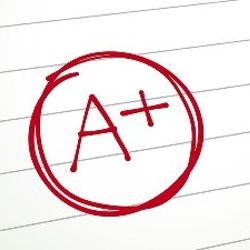# Watch Video Lecture Segment: 11 Problem Solving with Repetition. There are 3 problems solved. Test Scores, Gas Mileage, and Factorials. Type along with the video, pausing where necessary. Get each of these programs to work. Walk through the code in the dUOPOnlineTutor
(Not rated)
(Not rated)
Chat

COP 1000C Intro Programming Concepts. DR Archibald Videos

• Watch Video Lecture Segment 10 Mixed Type Expressions. Near the end of Segment 10 there are some practice exercises. This is Slide 10.7. Try them with a pencil. Then write a C program to verify your answers.
•  Watch Video Lecture Segment: 11 Problem Solving with Repetition. There are 3 problems solved. Test Scores, Gas Mileage, and Factorials. Type along with the video, pausing where necessary. Get each of these programs to work. Walk through the code in the debugger.

3- Include the use of ++, += in a program that achieves the following using loops. (You can do these in one program or each one in a small program, it doesn’t matter)

A-  Create a loop that will output all the multiples of 5 that are greater than zero and less than 60 (do not include 60). 5, 10, 15, 20, 25, 30, 35, 40, 45, 50, 55

B-  Create a loop that will output all the numbers less than 200 that are evenly divisible by both 2 and 7. 14 28 … C. Create a loop that will calculate the sum of the multiples of 8 that are between 100 and 500. Output the sum only. D. Create a loop that will output the sum of all odd numbers between 20 and 100.

• 5 years ago
A+ Solution
NOT RATED

Purchase the answer to view it

•10328666_1.zip